# JP3652518B2 - SIMD type arithmetic unit and arithmetic processing unit - Google Patents

## Info

Publication number
JP3652518B2
JP3652518B2 JP21702798A JP21702798A JP3652518B2 JP 3652518 B2 JP3652518 B2 JP 3652518B2 JP 21702798 A JP21702798 A JP 21702798A JP 21702798 A JP21702798 A JP 21702798A JP 3652518 B2 JP3652518 B2 JP 3652518B2
Authority
JP
Japan
Prior art keywords
flag
data
stored
unit
register
Prior art date
Legal status (The legal status is an assumption and is not a legal conclusion. Google has not performed a legal analysis and makes no representation as to the accuracy of the status listed.)
Expired - Fee Related
Application number
JP21702798A
Other languages
Japanese (ja)
Other versions
JP2000047998A (en
Inventor

Original Assignee

Priority date (The priority date is an assumption and is not a legal conclusion. Google has not performed a legal analysis and makes no representation as to the accuracy of the date listed.)
Filing date
Publication date
Application filed by 株式会社リコー filed Critical 株式会社リコー
Priority to JP21702798A priority Critical patent/JP3652518B2/en
Publication of JP2000047998A publication Critical patent/JP2000047998A/en
Application granted granted Critical
Publication of JP3652518B2 publication Critical patent/JP3652518B2/en
Anticipated expiration legal-status Critical
Expired - Fee Related legal-status Critical Current

• 230000000875 corresponding Effects 0.000 claims description 37
• 238000010586 diagrams Methods 0.000 description 18
• 238000000034 methods Methods 0.000 description 16
• 230000004048 modification Effects 0.000 description 9
• 238000006011 modification reactions Methods 0.000 description 9
• 238000006243 chemical reactions Methods 0.000 description 5
• 230000000694 effects Effects 0.000 description 4
• 238000007906 compression Methods 0.000 description 3
• 230000004044 response Effects 0.000 description 1

## Images

•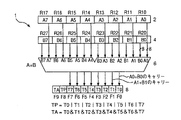•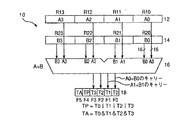•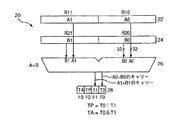•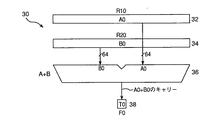••••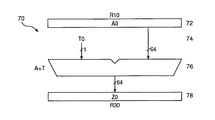•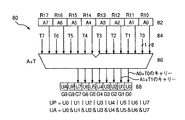•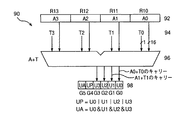•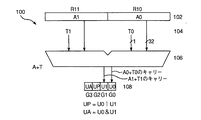•••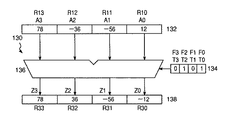••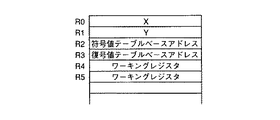••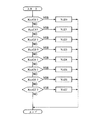•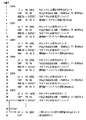•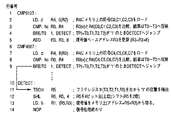•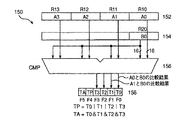## Classifications

• GPHYSICS
• G06COMPUTING; CALCULATING; COUNTING
• G06FELECTRIC DIGITAL DATA PROCESSING
• G06F9/00Arrangements for program control, e.g. control units
• G06F9/06Arrangements for program control, e.g. control units using stored programs, i.e. using an internal store of processing equipment to receive or retain programs
• G06F9/30Arrangements for executing machine instructions, e.g. instruction decode
• G06F9/30094Condition code generation, e.g. Carry, Zero flag
• GPHYSICS
• G06COMPUTING; CALCULATING; COUNTING
• G06FELECTRIC DIGITAL DATA PROCESSING
• G06F9/00Arrangements for program control, e.g. control units
• G06F9/06Arrangements for program control, e.g. control units using stored programs, i.e. using an internal store of processing equipment to receive or retain programs
• G06F9/30Arrangements for executing machine instructions, e.g. instruction decode
• G06F9/30003Arrangements for executing specific machine instructions
• G06F9/30007Arrangements for executing specific machine instructions to perform operations on data operands
• G06F9/3001Arithmetic instructions
• G06F9/30014Arithmetic instructions with variable precision
• GPHYSICS
• G06COMPUTING; CALCULATING; COUNTING
• G06FELECTRIC DIGITAL DATA PROCESSING
• G06F9/00Arrangements for program control, e.g. control units
• G06F9/06Arrangements for program control, e.g. control units using stored programs, i.e. using an internal store of processing equipment to receive or retain programs
• G06F9/30Arrangements for executing machine instructions, e.g. instruction decode
• G06F9/30003Arrangements for executing specific machine instructions
• G06F9/30007Arrangements for executing specific machine instructions to perform operations on data operands
• G06F9/30021Compare instructions, e.g. Greater-Than, Equal-To, MINMAX
• GPHYSICS
• G06COMPUTING; CALCULATING; COUNTING
• G06FELECTRIC DIGITAL DATA PROCESSING
• G06F9/00Arrangements for program control, e.g. control units
• G06F9/06Arrangements for program control, e.g. control units using stored programs, i.e. using an internal store of processing equipment to receive or retain programs
• G06F9/30Arrangements for executing machine instructions, e.g. instruction decode
• G06F9/30003Arrangements for executing specific machine instructions
• G06F9/30007Arrangements for executing specific machine instructions to perform operations on data operands
• G06F9/30029Logical and Boolean instructions, e.g. XOR, NOT
• GPHYSICS
• G06COMPUTING; CALCULATING; COUNTING
• G06FELECTRIC DIGITAL DATA PROCESSING
• G06F9/00Arrangements for program control, e.g. control units
• G06F9/06Arrangements for program control, e.g. control units using stored programs, i.e. using an internal store of processing equipment to receive or retain programs
• G06F9/30Arrangements for executing machine instructions, e.g. instruction decode
• G06F9/30003Arrangements for executing specific machine instructions
• G06F9/30007Arrangements for executing specific machine instructions to perform operations on data operands
• G06F9/30036Instructions to perform operations on packed data, e.g. vector operations

## Description


BACKGROUND OF THE INVENTION
The present invention relates to an arithmetic unit using a SIMD (Single Instruction Multiple Data) system and an arithmetic processing device (hereinafter referred to as a CPU) including the arithmetic unit.

[Prior art]
There is a SIMD system as a system for processing a plurality of data in parallel in a CPU. In the SIMD system, a plurality of operations are controlled in parallel by a single operation instruction in an arithmetic unit in the CPU. Further, there are advantages that the instruction supply device and the instruction control device can be shared and the processing execution time can be shortened.

[Problems to be solved by the invention]
On the other hand, in the SIMD type arithmetic unit, the calculation target data differs depending on the arithmetic unit, but the processing functions of the plurality of arithmetic operations are the same. That is, different processing cannot be performed for each operation unit. For example, it is difficult to replace only the data of the matching calculation with “0”, judging from the result of comparison calculation with a certain data for a certain data group.

In the SIMD method, one arithmetic unit is assigned to one arithmetic unit for processing, and a plurality of arithmetic units are generally used. However, this requires an unreasonably large circuit scale depending on the size of the arithmetic data. Sometimes. For example, even when there are many operations for 16-bit data, and in rare cases it is necessary to perform an operation process for 64-bit data, the CPU must have an arithmetic unit with the maximum data width up to the maximum number of parallel circuits. The device scale may not be used effectively.

An object of the present invention is to provide an SIMD type arithmetic unit and an arithmetic processing device that selectively execute subsequent processing according to a condition flag corresponding to a calculation result of a calculation unit.

The present invention also provides an arithmetic unit and an arithmetic processing unit for selectively executing subsequent processing in units of arithmetic data, and an arithmetic unit capable of handling the maximum data width even when a large amount of short bit data is processed in parallel. It is another object to make it possible to have a circuit scale that can be used effectively without necessarily providing up to the maximum number of parallel processes of data.

[Means for Solving the Problems]
First of the present invention one Is a SIMD type arithmetic unit having two input means and one output means, wherein the first input means has the same configuration as that of the arithmetic unit of the first form, The second input means uses the output means in the arithmetic unit of the first form. Therefore, using the data stored in each data storage section of the first input means and the corresponding condition flag stored in each flag storage section of the second input means, a set of each data and condition flag is simultaneously used. The common calculation is performed, and the calculation result is stored in the output means.

First of the present invention two Is a SIMD type arithmetic unit having at least one input means and one output means, in which the input means and the output means are of a predetermined bit length and bits of data to be stored It has a data storage unit whose number and bit length change according to the length. This SIMD type arithmetic unit stores data obtained as a result of performing a common operation on each data simultaneously using the data stored in each data storage unit of the input means in the data storage unit of the corresponding output means. However, in the SIMD type arithmetic unit of the present invention, each flag storage unit of the output means for storing the condition flag group output by the arithmetic unit of the first form is each data on the input means of the third form. When the data stored in each data storage unit of the input means is calculated, the data is stored for each data depending on the contents of the condition flag stored in each flag storage unit corresponding to the data storage unit. A condition is given to the operation.

First of the present invention three The form of The computing unit of the first form and the computing unit of the second form It is CPU provided with.

DETAILED DESCRIPTION OF THE INVENTION
Hereinafter, embodiments of the present invention will be described with reference to the accompanying drawings. A SIMD type arithmetic unit (hereinafter referred to as an arithmetic unit) according to the first embodiment of the present invention is shown in FIG. The computing unit 1 includes a first input register 2, a second input register 4, a computing unit 6, and an output register 8. The bit length of the two input registers 2 and 4 is 64 bits.

In the computing unit 1 shown in FIG. 1, each of the first and second input registers 2 and 4 includes eight data storage units R10 to R17 and R20 to R27 each having a bit length of 8 bits. Predetermined calculation data A0 to A7 and B0 to B7 can be stored in the storage unit. The output register 8 has ten flag storage units F0 to F9 each having a bit length of 1 bit, and each flag storage unit can store a predetermined flag (T0 to T7, TP, TA). .

In this computing unit 1, the input data A0 to A7 stored in the data storage units R10 to R17 of the first input register 2 and the input data stored in the data storage units R20 to R27 of the second input register 4 are used. A calculation common to each data set is simultaneously performed in the calculation unit 6 using each of B0 to B7, and flags T0 to T7 (0 or 1) corresponding to the calculation result are flag storage units F0 to F0 of the output register 8. Stored in F7. The flag storage unit F8 of the output register 8 stores a flag TP (0 or 1) corresponding to the result of the logical sum operation of the output flags T0 to T7 stored in the flag storage units F0 to F7. On the other hand, the flag TA (0 or 1) corresponding to the result of the logical product operation of the output flags T0 to T7 stored in the flag storage units F0 to F7 is stored in the data storage unit F9 of the output register.

The flags T0 to T7 stored in the flag storage units F0 to F7 of the output register 8 will be specifically described. For example, when two input data A0 and B0 are added by the arithmetic unit 6, if the result obtained by adding these data exceeds 8 bits (that is, when a carry occurs), the corresponding output flag is stored. The flag 1 is stored in the part F0. Conversely, when the addition result is 8 bits or less (that is, when no carry occurs), the flag 0 is stored in the corresponding output data storage unit F0.

FIG. 2 shows a modification of the arithmetic unit shown in FIG. Each of the first and second input registers 12 and 14 includes four data storage units R10 to R13 and R20 to R23 each having a bit length of 16 bits. Each data storage unit has predetermined operation data A0. -A3 and B0-B3 can be stored. The output register 18 has six flag storage units F0 to F5 having a bit length of 1 bit, and each flag storage unit can store predetermined flags (T0 to T3, TP, TA). . Here, the flags T0 to T3 stored in the flag storage units F0 to F3 of the output register 18 are obtained and stored in the same manner as the flags T0 to T7 of the arithmetic unit 1 of the embodiment of FIG. Although the flag TP is stored in the flag storage unit F4 of the output register 18, the logical sum operation of the output flags T0 to T3 stored in the flag storage units F0 to F3 is substantially the same as the embodiment of FIG. It corresponds to the result of. Similarly, the flag TA is stored in the flag storage unit F5 of the output register 18 and corresponds to the result of the logical product operation of the output flags T0 to T3 stored in the flag storage units F0 to F3.

FIG. 3 also shows a modification of the arithmetic unit shown in FIG. Each of the first and second input registers 22 and 24 includes two data storage units R10 to R11 and R20 to R21 each having a bit length of 32 bits. Each data storage unit has predetermined operation data A0. -A1 and B0-B1 can be stored. The output register 28 has four flag storage units F0 to F3 having a bit length of 1 bit, and each flag storage unit can store a predetermined flag (T0 to T1, TP, TA). . Here, the flags T0 to T1 stored in the flag storage units F0 to F1 of the output register 28 are obtained and stored in the same manner as the flags T0 to T7 of the arithmetic unit 1 of the embodiment of FIG. Although the flag TP is stored in the flag storage unit F2 of the output register 28, the logical sum operation of the output flags T0 to T1 stored in the flag storage units F0 to F1 is substantially the same as the embodiment of FIG. It corresponds to the result of. Similarly, the flag TA is stored in the flag storage unit F3 of the output register 28, and corresponds to the result of the logical product operation of the output flags T0 to T1 stored in the flag storage units F0 to F1.

FIG. 4 also shows a modification of the arithmetic unit shown in FIG. Each of the first and second input registers 32 and 34 is composed of one data storage unit R10 and R20 having a bit length of 64 bits, and predetermined operation data A0 and B0 are stored in each data storage unit. I can do it. The output register 38 has one flag storage unit F0 having a bit length of 1 bit, and a predetermined flag T0 can be stored in the flag storage unit. Here, the flag T0 stored in the flag storage unit F0 of the output register 38 is obtained and stored in the same manner as the flags T0 to T7 of the arithmetic unit 1 of the embodiment of FIG.

When a flag (hereinafter referred to as a condition flag) corresponding to the operation result output as described above by these arithmetic units is used, different processing can be performed for each operation unit in subsequent processing. Further, conditional branch processing by the condition flag can be performed.

If a flag (hereinafter referred to as a conditional OR flag) TP corresponding to the result of the OR operation of the condition flags output as described above by these arithmetic units is used, conditional branch processing by the conditional OR flag is used. Is possible. Similarly, by using a flag TA (hereinafter referred to as “conditional logical product flag”) TA corresponding to the result of the logical product operation of the condition flag, conditional branch processing by the conditional logical product flag becomes possible.

In the form of FIG. 1, only one arithmetic unit having an input register having a bit length of 64 bits is prepared, and eight operations can be performed in parallel within the single arithmetic unit. There is no need to prepare eight arithmetic units having a bit length input register. As a result, a small circuit scale can be realized. The same applies not only to the embodiment of FIG. 1 but also to FIGS.

1, 2, 3, and 4, the carry corresponding to the carry is shown as the flag corresponding to the result of the operation, but in addition to this, an overflow flag corresponding to the overflow of the operation result, It may be a zero flag corresponding to the operation result “0”, a negative flag corresponding to the operation result being negative, or the like.

FIG. 5 shows an arithmetic unit according to the second embodiment of the present invention. The arithmetic unit 40 includes a first input register 42, a second input register 44, an arithmetic unit 46, and an output register 48. Here, the second input register 44 is the first embodiment. An output register (hereinafter referred to as a flag register) 8 in the arithmetic unit 1 in the embodiment, and condition flags T0 to T7 stored in the flag register 8 are input data, that is, calculation target data. The bit length of the first input register 42 and the output register 48 is 64 bits.

In the arithmetic unit 40 of FIG. 5, the first input register 42 includes eight data storage units R10 to R17 having a bit length of 8 bits, and predetermined arithmetic data A0 to A7 are stored in each data storage unit. Can be stored. The second input register 44, that is, the flag register 8 is composed of at least eight flag storage units F0 to F7 having a bit length of 1 bit, and each flag storage unit includes an arithmetic unit according to the first embodiment. 1 stores condition flags T0 to T7 as output data. The output register 48 includes eight data storage units R30 to R37 having a bit length of 8 bits, and predetermined operation result data Z0 to Z7 can be stored in each data storage unit.

In the arithmetic unit 40, the input data A0 to A7 stored in the data storage units R10 to R17 of the first input register 42 and the flag storage units F0 to F7 of the second input register 44 (that is, the flag register 8). Using the condition flags T0 to T7 stored in the output unit 48, the operation unit 46 simultaneously performs a common operation for each data and condition flag set, and the operation results Z0 to Z7 are obtained as data storage units R30 to R30 of the output register 48. Stored in R37. For example, when two input data A0 and T0 are added by the calculation unit 46, the result Z0 obtained by adding these data is stored in the output data storage unit R30.

FIG. 6 shows a modification of the arithmetic unit shown in FIG. The first input register 52 is composed of four data storage units R10 to R13 having a bit length of 16 bits, and predetermined operation data A0 to A3 can be stored in each data storage unit. The second input register 54, that is, the flag register 18 is composed of at least four flag storage units F0 to F3 having a bit length of 1 bit, and each flag storage unit includes the arithmetic unit in the first form. 10 stores condition flags T0 to T3 as output data. The output register 58 is composed of four data storage units R30 to R33 having a bit length of 16 bits, and predetermined operation result data Z0 to Z3 can be stored in each data storage unit.

In the computing unit 50, the input data A0 to A3 stored in the data storage units R10 to R13 of the first input register 52 and the flag storage units F0 to F3 of the second input register 54 (that is, the flag register 18). Using the condition flags T0 to T3 stored in the same, the calculation unit 56 simultaneously performs a common calculation for each data and condition flag set, and the calculation results Z0 to Z3 are output to the data storage units R30 to R30 of the output register 58. Stored in R33. For example, when two input data A0 and T0 are added by the calculation unit 56, the result Z0 obtained by adding these data is stored in the output data storage unit R30.

FIG. 7 also shows a modification of the arithmetic unit shown in FIG. The first input register 62 is composed of two data storage units R10 to R11 having a bit length of 32 bits, and predetermined operation data A0 to A1 can be stored in each data storage unit. The second input register 64, that is, the flag register 28 is composed of at least two flag storage units F0 to F1 each having a bit length of 1 bit, and each flag storage unit includes the arithmetic unit according to the first embodiment. 20 stores condition flags T0 to T1 as output data. The output register 68 is composed of two data storage units R30 to R31 having a bit length of 32 bits, and predetermined operation result data Z0 to Z1 can be stored in each data storage unit.

In the arithmetic unit 60, the input data A0 to A1 stored in the data storage units R10 to R11 of the first input register 62 and the flag storage units F0 to F1 of the second input register 64 (that is, the flag register 28). Using the condition flags T0 to T1 stored in each of them, the calculation unit 66 simultaneously performs a common calculation for each data and condition flag set, and the calculation results Z0 to Z1 are stored in the data storage units R30 to R30 of the output register 68. Stored in R31. For example, when two input data A0 and T0 are added by the arithmetic unit 66, a result Z0 obtained by adding these data is stored in the output data storage unit R30.

FIG. 8 also shows a modification of the arithmetic unit shown in FIG. The first input register 72 includes one data storage unit R10 having a bit length of 64 bits, and can store predetermined calculation data A0. The second input register 74, that is, the flag register 38 is composed of at least one flag storage unit F0 having a bit length of 1 bit, and is a condition flag serving as output data in the arithmetic unit 30 in the first embodiment. T0 is stored. The output register 78 is composed of one data storage unit R30 having a bit length of 64 bits, and can store predetermined calculation result data Z0.

In this computing unit 70, the input data A0 stored in the data storage section R10 of the first input register 72 and the condition flag T0 stored in the flag storage section F0 of the second input register 74 (that is, flag register) are obtained. The operation unit 76 performs an operation, and the operation result Z0 is stored in the data storage unit R30 of the output register 78.

According to the arithmetic unit configured as described above, it becomes easy to reflect the result of the SIMD type operation executed in advance in the subsequent SIMD type operation in the unit of calculation.

In the form of FIG. 5, only one arithmetic unit having an input register having a 64-bit bit length is prepared, and eight operations can be performed in parallel within the single arithmetic unit. There is no need to prepare eight arithmetic units having a bit length input register. As a result, a small circuit scale can be realized. The same applies not only to the embodiment of FIG. 5 but also to FIGS.

FIG. 9 shows an arithmetic unit according to the third embodiment of the present invention. The computing unit 80 includes a first input register 82, a second input register 84, a computing unit 86, and an output register 88. In this configuration, the first embodiment is combined with the second embodiment of the present invention. That is, the second input register 84 is the same as that of the second embodiment and is the flag register 8 in the arithmetic unit 1 in the first embodiment, but the output register 88 is the same as that of the first embodiment. Similar to the embodiment, the condition flag corresponding to the operation result, the condition logical sum flag and the condition logical product flag determined by the contents of the condition flag are stored.

In the arithmetic unit 80 of FIG. 9, the first input register 82 includes eight data storage units R11 to R17 having a bit length of 8 bits, and predetermined arithmetic data A0 to A7 are stored in each data storage unit. Can be stored. The second input register 84, that is, the flag register 8 is composed of at least eight flag storage units F0 to F7 having a bit length of 1 bit, and each flag storage unit includes the arithmetic unit in the first form. 1 stores condition flags T0 to T7 as output data. The output register 88 has ten flag storage units G0 to G9 having a bit length of 1 bit, and each flag storage unit can store predetermined flags (U0 to U7, UP, UA). .

In this computing unit 80, the input data A0 to A7 stored in the data storage units R10 to R17 of the first input register 82 and the flag storage units R20 to R27 of the second input register 84 (that is, the flag register 8). The calculation unit 86 simultaneously performs a common calculation for each data and condition flag set using the condition flags T0 to T7 stored in the flag, and flags U0 to U7 (0 or 1) corresponding to the calculation result are obtained. It is stored in the flag storage units G0 to G7 of the output register 88. The flag storage unit G8 of the output register 88 stores a flag UP (0 or 1) corresponding to the result of the logical sum operation of the output flags U0 to U7 stored in the flag storage units G0 to G7. On the other hand, the data storage unit G9 of the output register stores a flag UA (0 or 1) corresponding to the result of the logical product operation of the output flags U0 to U7 stored in the flag storage units G0 to G7.

In FIG. 9, the flags U0 to U7 stored in the flag storage units G0 to G7 of the output register 88 are carry flags indicating carry as in the output flag of the arithmetic unit 1 of the first embodiment. .

FIG. 10 shows a modification of the arithmetic unit shown in FIG. The first input register 92 is composed of four data storage units R10 to R13 having a bit length of 16 bits, and predetermined operation data A0 to A3 can be stored in each data storage unit. The second input register 94, that is, the flag register 18 is composed of at least four flag storage units F0 to F3 having a bit length of 1 bit, and each flag storage unit includes the arithmetic unit in the first form. 10 stores condition flags T0 to T3 as output data. The output register 98 has six flag storage units G0 to G5 having a bit length of 1 bit, and each flag storage unit can store predetermined flags (U0 to U3, UP, UA). Here, the flags U0 to U3 stored in the flag storage units G0 to G3 of the output register 98 are obtained and stored in the same manner as the flags U0 to U7 of the arithmetic unit 80 in the embodiment of FIG. Although the flag UP is stored in the flag storage unit G4 of the output register 98, the logical sum operation of the output flags U0 to U3 stored in the flag storage units G0 to G3 is similar to the embodiment of FIG. It corresponds to the result of. Similarly, a flag UA is stored in the flag storage unit G5 of the output register 98, and corresponds to the result of the logical product operation of the output flags U0 to U3 stored in the flag storage units G0 to G3.

FIG. 11 also shows a modification of the arithmetic unit shown in FIG. The first input register 102 includes two data storage units R10 to R11 having a bit length of 32 bits, and predetermined operation data A0 to A1 can be stored in each data storage unit. The second input register 104, that is, the flag register 28 is composed of at least two flag storage units F0 to F1 having a bit length of 1 bit, and each flag storage unit includes the arithmetic unit according to the first embodiment. 20 stores condition flags T0 to T1 as output data. The output register 108 has four flag storage units G0 to G3 having a bit length of 1 bit, and each flag storage unit can store a predetermined flag (U0 to U1, UP, UA). Here, the flags U0 to U1 stored in the flag storage units G0 to G1 of the output register 108 are obtained and stored in the same manner as the flags U0 to U7 of the arithmetic unit 80 in the embodiment of FIG. Although the flag UP is stored in the flag storage unit G2 of the output register 108, the logical sum operation of the output flags U0 to U1 stored in the flag storage units G0 to G1 is similar to the embodiment of FIG. It corresponds to the result of. Similarly, the flag storage unit G3 of the output register 108 stores a flag UA, which corresponds to the result of the logical product operation of the output flags U0 to U1 stored in the flag storage units G0 to G1.

FIG. 12 also shows a modification of the arithmetic unit shown in FIG. The first input register 112 is composed of one data storage unit R10 having a 64-bit bit length, and can store predetermined calculation data A0. The second input register 114, that is, the flag register 38 is composed of at least one flag storage unit F0 having a bit length of 1 bit, and is a condition flag serving as output data in the arithmetic unit 30 in the first embodiment. T0 is stored. The output register 118 has one flag storage unit G0 having a bit length of 1 bit, and a predetermined flag U0 can be stored in the flag storage unit. Here, the flag U0 stored in the flag storage unit G0 of the output register 118 is obtained and stored in the same manner as the flags U0 to U7 of the arithmetic unit 80 in the embodiment of FIG.

According to the arithmetic unit configured as described above, it becomes easy to reflect the result of the SIMD type operation executed in advance in the subsequent SIMD type operation in the unit of calculation.

In the form of FIG. 9, only one arithmetic unit having an input register having a 64-bit bit length is prepared, and eight operations can be performed in parallel within the single arithmetic unit. There is no need to prepare eight arithmetic units having long input registers. As a result, a small circuit scale can be realized. The same applies to FIG. 10 and FIG. 11 as well as the configuration of FIG.

FIG. 13 shows an arithmetic unit according to the fourth embodiment of the present invention. The computing unit 120 includes an input register 122, a computing unit 126, and an output register 128. The bit length of the input register 122 and the output register 128 is 64 bits.

In the arithmetic unit 120 of FIG. 13, the input register 122 is composed of four data storage units R10 to R13 having a bit length of 16 bits, and predetermined arithmetic data A0 to A3 can be stored in each data storage unit. It is like that. The output register 128 is composed of four data storage units R30 to R33 having a bit length of 16 bits, and predetermined operation result data Z0 to Z3 can be stored in each data storage unit.

In this computing unit 120, the computation unit 126 simultaneously performs a common computation on each data using the input data A0 to A3 stored in each of the data storage units R10 to R13 of the input register 122. Each of the condition flags T0 to T3 stored in the flag register 18 in the computing unit 10 in the first embodiment gives a condition to each computation in the computing unit 126. The calculation results Z0 to Z3 are stored in the data storage units R30 to R33 of the output register 128.

A specific example of the arithmetic unit of the embodiment of FIG. 13 is shown in FIG. FIG. 14 shows how the four data A0 to A3 stored in the input register 132 are code-converted according to the values of the condition flags T0 to T3. Since A0 is “12” and the corresponding condition flag T0 is “1”, code conversion is performed, and the operation result data Z0 becomes “−12”. Since A1 is “−56” and the corresponding condition flag T1 is “0”, no sign conversion is performed, and the operation result data Z1 becomes “−56” as it is. The same conversion is performed for A2 and A3.

According to the arithmetic unit configured as described above, it is possible to selectively execute processing on arithmetic data that satisfies (or does not satisfy) the condition, based on the result of the SIMD type arithmetic executed previously.

In the form of FIG. 13 and FIG. 14, only one arithmetic unit having an input register having a bit length of 64 bits is prepared, and four arithmetic operations can be performed in parallel in the single arithmetic unit. Therefore, it is not necessary to prepare four arithmetic units having input registers having the same bit length. As a result, a small circuit scale can be realized.

FIG. 15 shows an arithmetic unit according to the fifth embodiment of the present invention. The computing unit 140 includes a first input register 142, a second input register 144, a computing unit 146, and an output register 148. This configuration is substantially the same configuration as the computing unit 10 of the first embodiment. However, the difference is that the second input register 144 has only one data storage unit B0.

The first input register 142 includes eight data storage units R10 to R17 having a bit length of 8 bits, and each data storage unit can store predetermined calculation data A0 to A7. The second input register 144 is composed of one data storage unit R20 having a bit length of 8 bits, and can store predetermined operation data B0 therein. The output register 148 has ten flag storage units F0 to F9 having a bit length of 1 bit, and each flag storage unit can store a predetermined flag (T0 to T7, TP, TA). .

In this computing unit 140, the input data A0 to A7 stored in the data storage units R10 to R17 of the first input register 142 and the input data stored in one data storage unit R20 of the second input register 144 are stored. A calculation common to each data set is simultaneously performed in the calculation unit 146 using each of B0, and flags T0 to T7 (0 or 1) corresponding to the calculation result are stored in the flag storage units F0 to F7 of the output register 148. Stored. The flag storage unit F8 of the output register 148 stores a flag TP (0 or 1) corresponding to the result of the logical sum operation of the output flags T0 to T7 stored in the flag storage units F0 to F7. On the other hand, the data storage unit F9 of the output register 148 stores a flag TA (0 or 1) corresponding to the result of the logical product operation of the output flags T0 to T7 stored in the flag storage units F0 to F7.

In FIG. 15, flags T0 to T7 stored in the flag storage units F0 to F7 of the output register 148 are carry flags indicating carry, similar to the output flag of the arithmetic unit 1 of the first embodiment. .

According to the arithmetic unit configured as described above, not only the effect obtained in the case of the arithmetic unit 10 of the first embodiment but also the same data in advance when it is desired to add the same data to a plurality of data. There is an inherent advantage that the trouble of arranging B0 in parallel with B1 to B7 can be saved, contributing to speedup.

In the above description related to the arithmetic unit of the third embodiment, the output register output in advance by the arithmetic unit of the first embodiment is used as the second input register. The means used as the register may be an output register that is output in advance by the arithmetic unit of the third embodiment. Similarly, means used as a second input register in the arithmetic unit of the second embodiment and a register for giving a condition to the arithmetic operation in the arithmetic unit of the fourth embodiment are also the same as those of the third embodiment. The output register may be output in advance by the arithmetic unit.

Next, an example of a processing program using the result obtained by the arithmetic unit of the present invention will be shown. The example shown here performs code pattern matching. This is used when a large amount of data is compressed by code conversion based on the table shown in Table 1 and this compressed data is stored or communicated. When compressing certain data, conversion to a code having a smaller code amount than that data is performed. On the other hand, the compressed data is used after obtaining a decoded value from the compressed code, that is, expanding.

[Table 1]

The compression / decompression procedure by the encoding / decoding table of Table 1 is illustrated. If the data to be compressed is 'V5', the compressed code C5 is obtained from Table 1 based on this. On the other hand, when decompressing, the code that has been compressed and whose decoding value is unknown is compared with C0, C1, C2,... In Table 1, and the decoding value when they match is obtained from the table. In this example, when the code matches “C5”, the decoded value V5 at that time is obtained. One of the typical compression / decompression methods is variable length (Huffman) encoding / decoding, which is well known to those skilled in the art, and these are widely used for MPEG image compression / decompression and the like. Has been. An example of decompression processing for obtaining a decoded value from compressed data using the encoding / decoding table shown in Table 1 is shown below.

FIG. 16 shows a configuration of a register group used in the decompression process. X stored in the register R0 is a compressed code and y stored in the register R1 is a decoded value. The register R2 is a head (base) address on the memory where the code value table is stored, and the register R3 is a head (base) address on the memory where the decoded value table is stored. Both the register R4 and the register R5 are working registers. FIG. 17 shows how the code value table and the decoded value table are stored in the memory. In this example of decompression processing, both the code value and the decoded value are 16-bit (2 bytes) data. FIG. 18 shows a flowchart for obtaining a decoded value of the code code x whose decoding value is unknown based on the above conditions.

FIG. 19 shows an example of a program assembled in assembly language to obtain a decoded value according to the flowchart of FIG. 18, and the detailed processing contents are also shown in the drawing.

In FIG. 19, the second to fifth lines show a comparison between the first code C0 and the code code x on the encoding / decoding table of Table 1. In the second row, the first code value C0 of the code value table on the memory is stored in the register R4. In the third row, a comparison operation is performed between the register R4 that stores this code and the register R0 that stores the code code x whose decoding value is unknown, and as a result, if they match, a flag T0 ( 1 bit is output as T0 = '1'. This comparison operation is the first embodiment of the arithmetic unit showing an example of addition, and in particular, in FIG. 4, the addition operation and the comparison operation are replaced. If the signs match in the third row and '1' is stored in bit T0, branch processing is performed in accordance with the contents of T0 in the fourth row. The branch destination is the decoded value load sequence from the 30th row. If the signs do not match and '0' is stored in T0, the process does not branch at the fourth line, and proceeds to the fifth line.

In the fifth line, the decoded value table address R3 is incremented in response to the fact that the comparison operation did not match. Thereafter, generally, the comparison operation sequence as in the second to fifth rows is repeated while changing the comparison target code in order. Here, when the code code x whose decoding value is unknown is C5, a match with the code C5 is detected in the 29th line of FIG. 19, and the process branches to the decoding value loading sequence in the 30th line. In the above embodiment, when the code is C5, it is understood that 24 instructions need to be executed to finish the decoding process.

In the above example, one code is stored in each of the registers R0 and R4, a comparison operation process is performed, a flag T0 corresponding to the result is output, and a branch process is performed using the flag as a determination condition. A plurality of codes are stored in two input registers, arithmetic processing is performed by the SIMD method, a plurality of flags (T0, T1,... Tn) are output, and branch processing is performed using each flag as a determination condition. Is possible.

FIG. 20 further shows an example of a decryption processing program using a conditional OR flag. The second to fifth rows are comparison operation parts according to the SIMD system between the four code codes (codes C0, C1, C2, C3) and the code code x whose decoding value is unknown. In the second row, these four code codes (64 bits in total) are stored from the memory into the register R4, and in the third row, a comparison is made with the code code x whose decoding value is unknown. FIG. 21 shows the state of the arithmetic unit for this comparison arithmetic processing.

In FIG. 21, code codes C0 to C3 are stored in the data storage units A0 to A3 of the register Rs1, and a code code x whose decoding value is unknown is stored in the data storage unit B0 of the register Rs2. Here, the computing unit 140 of the fifth embodiment is applied. The result of each comparison operation is set in the condition flags T0 to T3, and the contents of the condition OR flag TP and the condition AND flag TA are set based on T0 to T3. These flags are stored in the corresponding flag storage unit of the flag register. Stored.

In the fourth line of FIG. 20, it is determined by the conditional OR flag TP whether or not a match has occurred among the four comparison operations. If there is a match, the process branches to the decoded value load sequence in the tenth and subsequent lines. . If there is no match, the base address R2 of the code table is incremented by 4 codes (8 bytes in total) for comparison with the next code code C4, C5, C6, C7. As shown in the example of FIG. 19, when the code x whose decoding value is unknown is C5, it is determined by the conditional OR flag TP that it matches any of C4, C5, C6, and C7 in the ninth row of FIG. 20. Detected and branch to the decoded value load sequence from the 10th row.

The “TSCH R5” instruction in the eleventh line searches for the flags T3, T2, T1, and T0 on the flag register from the left (upper) side, and stores the position of the first “1” on the register in R5. In this embodiment, since the decoded value is 2-byte data, the value of the coincidence position obtained in the 11th row is doubled in the 12th row, and the address increment R5 and the decoding table base address obtained immediately before in the 13th row. The decoded value is loaded into the register R1 using the addition value with R3 as an address, and the decoding process ends. In this example, when the unknown code x is C5, the number of instructions to be executed is 10, and the number of instructions to be executed is reduced to less than half compared to the 24 instructions in FIG. You can see that

In the above-described MPEG decoding process, since a large amount of such decoding process is performed, the speed-up effect according to the present invention is considerably large.

In the above example, the branch process is performed using the conditional OR flag as a determination condition. However, depending on the logic of the program, the branch process may be performed using the conditional AND flag as a determination condition for the branch process.

【The invention's effect】
As is apparent from the above description, the SIMD type arithmetic processing apparatus of the present invention has the following effects.

Using two data stored in the corresponding data storage units between the two input means, respectively, perform a common calculation at the same time, and store the condition flags corresponding to the respective calculation results in the corresponding flag storage unit of the output means In the SIMD type arithmetic unit of the present invention, a plurality of and common operations are simultaneously performed even in one-step instruction execution, and a plurality of conditions can be generated from these results. Less time is spent than generating conditions, leading to faster processing. Further, it becomes easy to search for an operation that satisfies (or does not satisfy) the condition. Furthermore, if a conditional OR flag, which is the logical sum of the condition flags, is output, it can be determined whether all of the plurality of operation data calculated at one time are unsatisfied with the condition, or whether at least one of the operation data is satisfied with the condition. Just check the sum flag. Similarly, if the conditional logical product flag is output, just check this conditional logical product flag to see if all of the multiple operation data calculated at once are satisfied with the condition, or whether at least one of them is unsatisfied with the condition. Prove.

In the above SIMD arithmetic unit, the second input means is at least equal to or longer than the bit length of the data storage section of the first input means, and equal in length to the data storage section of the first input means Each data set using the data stored in each data storage unit of the first input means and the data stored in one data storage unit of the second input means. When performing operations common to the two, it is advantageous in terms of processing speed and ease of instruction designation, particularly when a certain numerical value is added to a plurality of data.

In the SIMD arithmetic unit of the present invention in which one of the input means is the output means of the SIMD arithmetic unit, that is, the flag register, the result of the arithmetic operation executed previously can be reflected in the subsequent arithmetic operation.

In the SIMD arithmetic unit of the present invention, a condition flag stored in the flag register is associated with data stored in each data storage unit of the input means, and a condition is given to the operation according to the contents of the condition flag. Based on the result of the SIMD operation, it is possible to selectively execute processing only for data that satisfies (or does not satisfy) the condition. If the execution content of the operation data unit corresponding to the condition flag cannot be changed, only the data that satisfies the condition (or not) is extracted and processed, or the data that does not satisfy (or does not satisfy) the condition is followed. Therefore, it is necessary to devise such that the processing is not affected, and this is disadvantageous in terms of processing speed and ease of processing.

In a CPU having a conditional branch processing function that uses a condition flag generated by the SIMD arithmetic unit of the present invention as a judgment condition for branch processing, even if the calculation is executed at a time, individual processing is thereafter given by the result condition flag. Can do. Furthermore, the conditional branch processing function using the conditional logical sum flag or the conditional logical product flag as a judgment condition for the branch processing makes it possible to set individual processing depending on the contents of each flag.

The CPU having the function of digitizing the position of the flag storage unit storing “1” (or “0”) positioned at the highest (or lowest) position on the output means storing the condition flag satisfies the condition. It is possible to easily determine which operation is (or unsatisfactory). If this function is not available, each condition flag is checked for the presence of “1” (or “0”), and the first “1” (or “0”) condition flag is the position of the desired data. It is disadvantageous in terms of processing speed and ease, because the position must be digitized by the program.
[Brief description of the drawings]
FIG. 1 is an explanatory diagram (1) of condition flag generation by a SIMD calculator.
FIG. 2 is an explanatory diagram (2) of condition flag generation by a SIMD calculator.
FIG. 3 is an explanatory diagram (3) of condition flag generation by a SIMD calculator.
FIG. 4 is an explanatory diagram (4) of condition flag generation by a SIMD calculator.
FIG. 5 is an explanatory diagram (1) of a calculation by a SIMD calculator using a condition flag.
FIG. 6 is an explanatory diagram (2) of a calculation by a SIMD calculator using a condition flag.
FIG. 7 is an explanatory diagram (3) of a calculation by a SIMD calculator using a condition flag.
FIG. 8 is an explanatory diagram (4) of a calculation by a SIMD calculator using a condition flag.
FIG. 9 is an explanatory diagram (5) of calculation by a SIMD calculator using a condition flag.
FIG. 10 is an explanatory diagram (6) of a calculation by a SIMD calculator using a condition flag.
FIG. 11 is an explanatory diagram (7) of calculation by a SIMD calculator using a condition flag.
FIG. 12 is an explanatory diagram (8) of the calculation by the SIMD calculator using the condition flag.
FIG. 13 is an explanatory diagram of SIMD operation control using a condition flag bit.
FIG. 14 is a specific explanatory diagram of SIMD operation control by a condition flag bit.
FIG. 15 is an explanatory diagram of condition flag generation according to the present invention using a broadcast method.
FIG. 16 is an explanatory diagram of a register used in decompression processing.
FIG. 17 is an explanatory diagram of storage of a code value and a decoded value on a memory.
FIG. 18 is a flowchart for obtaining a decoded value of a code code x.
FIG. 19 is a program for obtaining a decoded value of a code code x.
FIG. 20 is a program for obtaining a decoded value of a code code x using a conditional OR flag.
FIG. 21 is an explanatory diagram of a comparison operation using a broadcast method in the program of FIG.
[Explanation of symbols]
DESCRIPTION OF SYMBOLS 1, 10, 20, 30 ... SIMD type | mold arithmetic unit, 2, 12, 22, 32 ... 1st input register, 4, 14, 24, 34 ... 2nd input register, 6, 16 , 26, 36... Arithmetic unit, 8, 18, 28, 38... Output register, 40, 50, 60, 70... SIMD type arithmetic unit, 42, 52, 62, 72. Input register 44, 54, 64, 74 ... second input register 46, 56, 66, 76 ... arithmetic unit, 48, 58, 68, 78 ... output register 80, 90, 100, 110 ... SIMD type arithmetic unit, 82, 92, 102, 112 ... first input register, 84, 94, 104, 114 ... second input register, 86, 96, 106, 116 ... Calculation unit, 88, 98, 108, 118 ... Output registers 120, 130 ... SIMD type arithmetic unit, 122, 132 ... input register, 126, 136 ... arithmetic unit, 128, 138 ... output register, 140 ... SIMD type arithmetic unit, 142. ..First input register, 144... Second input register, 146... Arithmetic unit, 148... Output register, Rs1.

## Claims (5)

1. An arithmetic unit having two input means and one output means,
The first input means has a data storage unit that has a predetermined bit length and whose number and bit length change according to the bit length of data to be stored,
The second input means has a flag storage part having a length of 1 bit up to the number of the data storage parts in the first input means, and the operation result in the preceding operation in each flag storage part Store the corresponding condition flag,
The data stored in each data storage section of the first input means and the condition flag stored in each flag storage section of the second input means corresponding thereto are used simultaneously for each data and condition flag set. An arithmetic unit that performs the above operation and stores it in output means.
2. An arithmetic unit having at least one input means and one output means,
The input means and the output means have a data storage unit that has a predetermined bit length, and the number and the bit length change according to the bit length of the data to be stored,
In an arithmetic unit for storing data obtained as a result of performing a common operation on each data simultaneously using data stored in each data storage unit of the input means in the data storage unit of the corresponding output means,
The operation control means has a flag storage part having a length of 1 bit up to the number of the data storage parts in the input means, and a condition flag corresponding to the operation result in the preceding operation is stored in each flag storage part. Store and
Each flag storage unit corresponds to each data storage unit of the input unit, and when data stored in each data storage unit of the input unit is used for calculation, each flag storage unit corresponding to the data storage unit A computing unit in which a condition is given to each data depending on the contents of the stored condition flag.
3. A central processing unit comprising the arithmetic unit according to claim 1.
4. A central processing unit comprising the arithmetic unit according to claim 2.
5. A central processing unit comprising the arithmetic unit according to claim 1 and the arithmetic unit according to claim 2.
JP21702798A 1998-07-31 1998-07-31 SIMD type arithmetic unit and arithmetic processing unit Expired - Fee Related JP3652518B2 (en)

## Priority Applications (1)

Application Number Priority Date Filing Date Title
JP21702798A JP3652518B2 (en) 1998-07-31 1998-07-31 SIMD type arithmetic unit and arithmetic processing unit

## Applications Claiming Priority (1)

Application Number Priority Date Filing Date Title
JP21702798A JP3652518B2 (en) 1998-07-31 1998-07-31 SIMD type arithmetic unit and arithmetic processing unit

## Publications (2)

Publication Number Publication Date
JP2000047998A JP2000047998A (en) 2000-02-18
JP3652518B2 true JP3652518B2 (en) 2005-05-25

# Family

## Family Applications (1)

Application Number Title Priority Date Filing Date
JP21702798A Expired - Fee Related JP3652518B2 (en) 1998-07-31 1998-07-31 SIMD type arithmetic unit and arithmetic processing unit

## Country Status (1)

JP (1) JP3652518B2 (en)

## Families Citing this family (9)

* Cited by examiner, † Cited by third party
Publication number Priority date Publication date Assignee Title
JP3857614B2 (en) 2002-06-03 2006-12-13 松下電器産業株式会社 Processor
JP3958662B2 (en) 2002-09-25 2007-08-15 松下電器産業株式会社 Processor
US7219213B2 (en) * 2004-12-17 2007-05-15 Intel Corporation Flag bits evaluation for multiple vector SIMD channels execution
EP1870803A4 (en) 2005-03-31 2008-04-30 Matsushita Electric Ind Co Ltd Processor
JP4716911B2 (en) * 2006-03-31 2011-07-06 日立アロカメディカル株式会社 Ultrasound diagnostic processor
JP2008071130A (en) * 2006-09-14 2008-03-27 Ricoh Co Ltd Simd type microprocessor
KR100863515B1 (en) 2006-10-13 2008-10-15 연세대학교 산학협력단 Method and Apparatus for decoding video signal
CN104011653B (en) * 2011-12-29 2017-09-15 英特尔公司 Packing data operation mask comparator processor, method, system
US20140281418A1 (en) * 2013-03-14 2014-09-18 Shihjong J. Kuo Multiple Data Element-To-Multiple Data Element Comparison Processors, Methods, Systems, and Instructions

## Also Published As

Publication number Publication date
JP2000047998A (en) 2000-02-18

## Similar Documents

Publication Publication Date Title
US8024394B2 (en) Dual mode floating point multiply accumulate unit
JP5005342B2 (en) Processor architecture that performs wide transform slice instructions
EP0655680B1 (en) Arithmetic and logic unit having a plurality of independent sections and a register for storing the status bits
JP3889069B2 (en) Programmable processor, method for performing digital signal processing using the programmable processor, and improvements thereof
TWI402766B (en) Graphics processor
US8261043B2 (en) SIMD merge-sort and duplicate removal operations for data arrays
US6240437B1 (en) Long instruction word controlling plural independent processor operations
EP0743593B1 (en) Replication of data
AU618142B2 (en) Tightly coupled multiprocessor instruction synchronization
US5206940A (en) Address control and generating system for digital signal-processor
US5606677A (en) Packed word pair multiply operation forming output including most significant bits of product and other bits of one input
US5596763A (en) Three input arithmetic logic unit forming mixed arithmetic and boolean combinations
TWI229287B (en) Single instruction multiple data (SIMD) processor capable of designating plural registers
US6941446B2 (en) Single instruction multiple data array cell
US4021655A (en) Oversized data detection hardware for data processors which store data at variable length destinations
US5847978A (en) Processor and control method for performing proper saturation operation
US5590350A (en) Three input arithmetic logic unit with mask generator
US5696954A (en) Three input arithmetic logic unit with shifting means at one input forming a sum/difference of two inputs logically anded with a third input logically ored with the sum/difference logically anded with an inverse of the third input
JP3729881B2 (en) Circuit and method for performing parallel addition and averaging
US5465224A (en) Three input arithmetic logic unit forming the sum of a first Boolean combination of first, second and third inputs plus a second Boolean combination of first, second and third inputs
US20130097408A1 (en) Conditional compare instruction
EP0656584B1 (en) Conditional memory store from a register pair
DE2724125C2 (en)
US8271571B2 (en) Microprocessor
JP4277042B2 (en) Arithmetic processing unit

## Legal Events

Date Code Title Description
A131 Notification of reasons for refusal

Free format text: JAPANESE INTERMEDIATE CODE: A131

Effective date: 20041124

A521 Written amendment

Free format text: JAPANESE INTERMEDIATE CODE: A523

Effective date: 20050120

TRDD Decision of grant or rejection written
A01 Written decision to grant a patent or to grant a registration (utility model)

Free format text: JAPANESE INTERMEDIATE CODE: A01

Effective date: 20050215

A61 First payment of annual fees (during grant procedure)

Free format text: JAPANESE INTERMEDIATE CODE: A61

Effective date: 20050223

R150 Certificate of patent or registration of utility model

Free format text: JAPANESE INTERMEDIATE CODE: R150

FPAY Renewal fee payment (event date is renewal date of database)

Free format text: PAYMENT UNTIL: 20090304

Year of fee payment: 4

FPAY Renewal fee payment (event date is renewal date of database)

Free format text: PAYMENT UNTIL: 20100304

Year of fee payment: 5

FPAY Renewal fee payment (event date is renewal date of database)

Free format text: PAYMENT UNTIL: 20110304

Year of fee payment: 6

FPAY Renewal fee payment (event date is renewal date of database)

Free format text: PAYMENT UNTIL: 20120304

Year of fee payment: 7

FPAY Renewal fee payment (event date is renewal date of database)

Free format text: PAYMENT UNTIL: 20130304

Year of fee payment: 8

FPAY Renewal fee payment (event date is renewal date of database)

Free format text: PAYMENT UNTIL: 20140304

Year of fee payment: 9

LAPS Cancellation because of no payment of annual fees